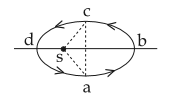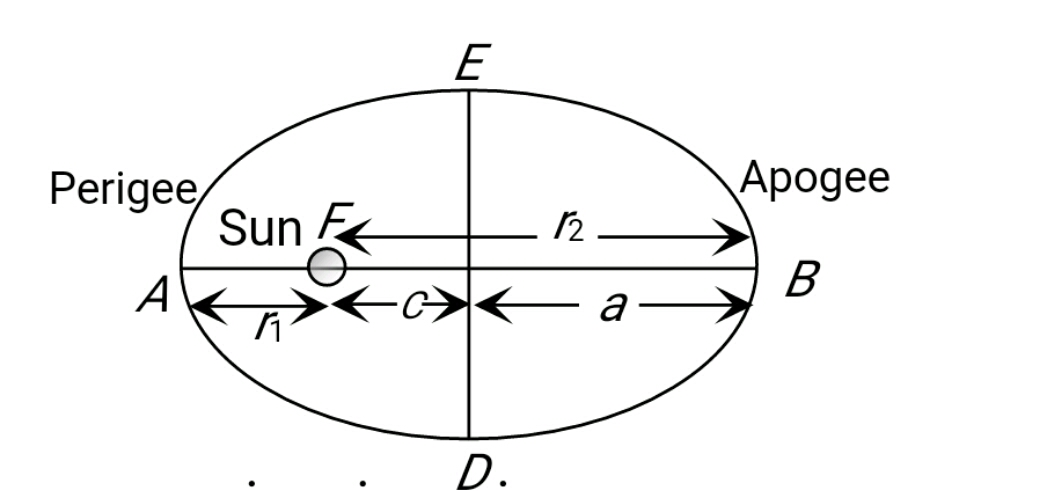#### Figure shows elliptical path abcd of a planet around the sun S such that the area of triangle csa isthe area of the ellipse. (See figure) With db as the semimajor axis, and ca as the semiminor axis.  If t1 is the time taken for planet to go over path abc and t2 for path taken over cda then :Option 1) t1 = t2 Option 2)  t1 = 2t2 Option 3)  t1 = 3t2 Option 4)  t1 = 4t2As we learnt in

Kepler's 3rd law -From fig.semi major AxisPerigee

- wherein

Known as law of periodsapogeeOption 1)

t1 = t2

Incorrect

Option 2)

t1 = 2t2

Incorrect

Option 3)

t1 = 3t2

Correct

Option 4)

t1 = 4t2

Incorrect

##### Posted by